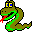#Boost C++ Libraries

...one of the most highly regarded and expertly designed C++ library projects in the world.#breadth_first_visit

```  template <class IncidenceGraph, class P, class T, class R>
typename graph_traits<IncidenceGraph>::vertex_descriptor s,
const bgl_named_params<P, T, R>& params);

template <class IncidenceGraph, class Buffer, class BFSVisitor, class ColorMap>
(const IncidenceGraph& g,
typename graph_traits<IncidenceGraph>::vertex_descriptor s,
Buffer& Q, BFSVisitor vis, ColorMap color)
```
This function is basically the same as breadth_first_search() except that the color markers are not initialized in the algorithm. The user is responsible for making sure the color for every vertex is white before calling the algorithm. With this difference, the graph type is only required to be an Incidence Graph instead of a Vertex List Graph. Also, this difference allows for more flexibility in the color property map. For example, one could use a map that only implements a partial function on the vertices, which could be more space efficient when the search only reaches a small portion of the graph.

### Parameters

IN: IncidenceGraph& g
A directed or undirected graph. The graph type must be a model of Incidence Graph.
Python: The parameter is named graph.
IN: vertex_descriptor s
The source vertex where the search is started.
Python: The parameter is named root_vertex.

### Named Parameters

IN: visitor(BFSVisitor vis)
A visitor object that is invoked inside the algorithm at the event-points specified by the BFS Visitor concept. The visitor object is passed by value .
Default: bfs_visitor<null_visitor>
Python: The parameter should be an object that derives from the BFSVisitor type of the graph.
UTIL/OUT: color_map(ColorMap color)
This is used by the algorithm to keep track of its progress through the graph. The type ColorMap must be a model of Read/Write Property Map and its key type must be the graph's vertex descriptor type and the value type of the color map must model ColorValue.
Default: get(vertex_color, g)
Python: The color map must be a vertex_color_map for the graph.
UTIL: buffer(Buffer& Q)
The queue used to determine the order in which vertices will be discovered. If a FIFO queue is used, then the traversal will be according to the usual BFS ordering. Other types of queues can be used, but the traversal order will be different. For example Dijkstra's algorithm can be implemented using a priority queue. The type Buffer must be a model of Buffer.
Default: boost::queue
Python: The buffer must derive from the Buffer type for the graph.

### Complexity

The time complexity is O(E).

### Visitor Event Points

• vis.examine_vertex(u, g)r is invoked in each vertex as it is removed from the queue.
• vis.examine_edge(e, g) is invoked on every out-edge of each vertex immediately after the vertex is removed from the queue.
• vis.tree_edge(e, g) is invoked (in addition to examine_edge()) if the edge is a tree edge. The target vertex of edge e is discovered at this time.
• vis.discover_vertex(u, g) is invoked the first time the algorithm encounters vertex u. All vertices closer to the source vertex have been discovered, and vertices further from the source have not yet been discovered.
• vis.non_tree_edge(e, g) is invoked (in addition to examine_edge()) if the edge is not a tree edge.
• vis.gray_target(e, g) is invoked (in addition to non_tree_edge()) if the target vertex is colored gray at the time of examination. The color gray indicates that the vertex is currently in the queue.
• vis.black_target(e, g) is invoked (in addition to non_tree_edge()) if the target vertex is colored black at the time of examination. The color black indicates that the vertex is no longer in the queue.
• vis.finish_vertex(u, g) is invoked after all of the out edges of u have been examined and all of the adjacent vertices have been discovered.